[TOC]

# go语言的31个坑

devs.cloudimmunity.com/gotchas-and…## 1.左大括号不能单独放一行 {

``````// 错误示例
func main()
{
println("www.topgoer.com是个不错的go语言中文文档")
}

// 等效于
func main();    // 无函数体
{
println("hello world")
}

// 正确示例
func main() {
println("Golang新手可能会踩的50个坑")
}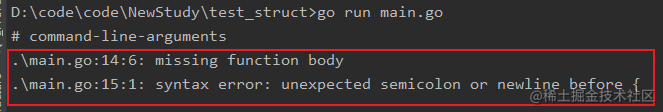## 2.不能使用简短声明来设置字段的值

struct 的变量字段不能使用` :=` 来赋值以使用预定义的变量来避免解决：

``````// 错误示例
package main

import "fmt"

type info struct {
result int
}

func work() (int, error) {
return 3, nil
}

func main() {
var data info
data.result, err := work() // error: non-name data.result on left side of :=
if err != nil{
fmt.Println(err)
return
}
fmt.Printf("info: %+v\n", data)
}

// 正确示例
func work() (int, error) {
return 3, nil
}

func main() {

tmp, err := work() // error: non-name data.result on left side of :=
if err != nil {
fmt.Println(err)
return
}
fmt.Printf("info: %+v\n", tmp)
}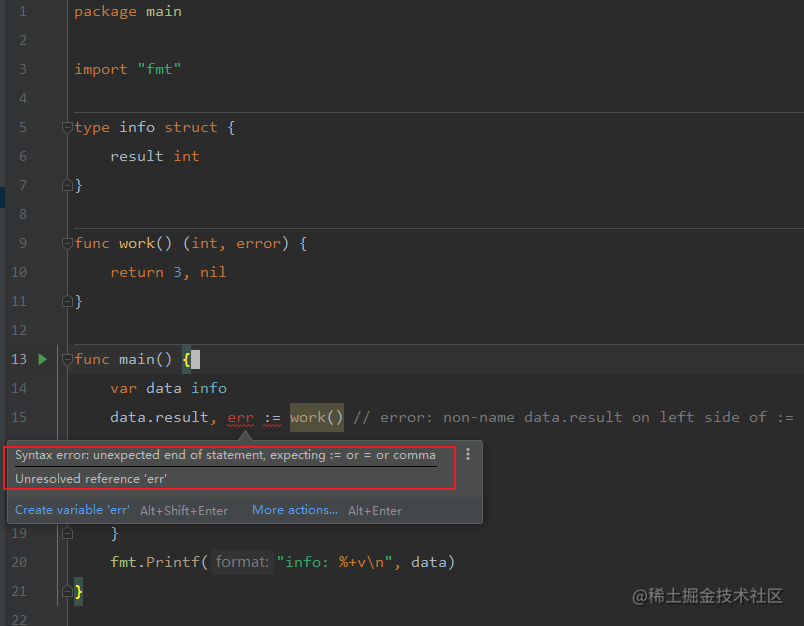## 3.不小心覆盖了变量

``````func main() {
x := 1
println(x)        // 1
{
println(x)    // 1
x := 2
println(x)    // 2    // 新的 x 变量的作用域只在代码块内部
}
println(x)        // 1
}

``````    > go tool vet -shadow main.go
main.go:9: declaration of "x" shadows declaration at main.go:5

``````    > \$GOPATH/bin/go-nyet main.go

## 4.显式类型的变量无法使用 nil 来初始化

nil 是 一下 6 种 类型变量的默认初始值。但声明时不指定类型，编译器也无法推断出变量的具体类型。

• interface

• function

• pointer

• map

• slice

• channel

``````// 错误示例
func main() {
var x = nil    // error: use of untyped nil
_ = x
}

// 正确示例
func main() {
var x interface{} = nil
_ = x
}

## 5.直接使用值为 nil 的 slice、map

• 允许对值为 nil 的 slice 添加元素

因为切片是实现方式是类似于c++ 的 vector，动态扩展内存的

• 对值为 nil 的 map添加元素则会造成运行时 panic

map的初始化必须分配好内存，否则直接报错

``````// map 错误示例
func main() {
var m map[string]int
m["one"] = 1        // error: panic: assignment to entry in nil map
// m := make(map[string]int)// map 的正确声明，分配了实际的内存
}

// slice 正确示例
func main() {
var s []int
s = append(s, 1)
}

func main() {
//m := map[string]int{}
m := make(map[string]int, 1)
m["one"] = 1
}

## 6.map 容量

``````// 错误示例
func main() {
m := make(map[string]int, 99)
println(cap(m))     // error: invalid argument m1 (type map[string]int) for cap
}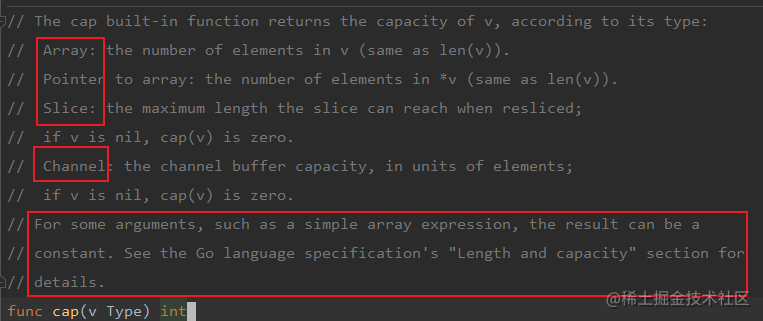• array
• pointer
• sliice
• channel

## 7.string 类型的变量值不能为 nil

``````// 错误示例
func main() {
var s string = nil    // cannot use nil as type string in assignment
if s == nil {    // invalid operation: s == nil (mismatched types string and nil)
s = "default"
}
}

// 正确示例
func main() {
var s string    // 字符串类型的零值是空串 ""
if s == "" {
s = "default"
}
}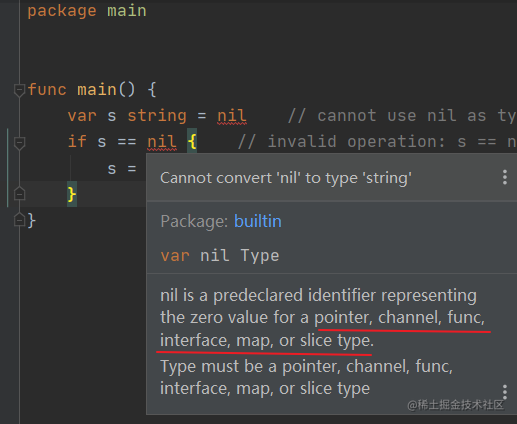• 指针
• 通道
• 函数
• 接口
• map
• 切片

## 8.Array 类型的值作为函数参数

Go 中，数组是值。作为参数传进函数时，传递的是数组的原始值拷贝，此时在函数内部是无法更新该数组的：

``````// 数组使用值拷贝传参
func main() {
x := int{3,4,5}

func(arr int) {
arr = 8
fmt.Println(arr)    // [8 4 5]
}(x)
fmt.Println(x)            // [3 4 5]    // 并不是你以为的 [8 4 5]
}

• 直接传递指向这个数组的指针类型
``````// 传址会修改原数据
func main() {
x := int{3,4,5}

func(arr *int) {
(*arr) = 8
fmt.Println(arr)    // &[8 4 5]
}(&x)
fmt.Println(x)    // [8 4 5]
}

• 直接使用 slice：即使函数内部得到的是 slice 的值拷贝，但依旧会更新 slice 的原始数据（底层 array）

因为slice是引用的方式传递

``````// 会修改 slice 的底层 array，从而修改 slice
func main() {
x := []int{1, 2, 3}
func(arr []int) {
arr = 7
fmt.Println(x)    // [8 4 5]
}(x)
fmt.Println(x)    // [8 4 5]
}

### golang中分为值类型和引用类型

• 值类型分别有

int系列、float系列、bool、string、数组和结构体

• 引用类型有：

• 值类型的特点是

变量直接存储值，内存通常在栈中分配

• 引用类型的特点是

变量存储的是一个地址，这个地址对应的空间里才是真正存储的值，内存通常在堆中分配

## 9.访问 map 中不存在的 key

Go 则会返回元素对应数据类型的零值，比如 nil、'' 、false 和 0，取值操作总有值返回，故不能通过取出来的值来判断 key 是不是在 map 中。

• 对于值类型：布尔类型为 `false`, 数值类型为 `0`，字符串为 `""`
• 数组和结构会递归初始化其元素或字段
• 其初始值取决于元素类型或字段
• 对于引用类型均为 `nil`，包括指针 pointer，函数 function，接口 interface，切片 slice，管道 channel，映射 map。

``````// 错误的 key 检测方式
func main() {
x := map[string]string{"one": "2", "two": "", "three": "3"}
if v := x["two"]; v == "" {
fmt.Println("key two is no entry")    // 键 two 存不存在都会返回的空字符串
}
}

// 正确示例
func main() {
x := map[string]string{"one": "2", "two": "", "three": "3"}
if _, ok := x["two"]; !ok {
fmt.Println("key two is no entry")
}
}

## 10.string 类型的值是常量，不可更改，可以使用rune来转换

• 英文字符串

string 类型的值是只读的二进制 byte slice，将 string 转为 []byte 修改后，再转为 string 即可

``````// 修改字符串的错误示例
func main() {
x := "text"
x = "T"        // error: cannot assign to x
fmt.Println(x)
}

// 修改示例
func main() {
x := "text"
xBytes := []byte(x)
xBytes = 'T'    // 注意此时的 T 是 rune 类型
x = string(xBytes)
fmt.Println(x)    // Text
}

• 中文字符串

一个 UTF8 编码的字符可能会占多个字节，比如汉字就需要 `3~4`个字节来存储，此时需要使用如下做法，使用 rune slice

将 string 转为 rune slice（此时 1 个 rune 可能占多个 byte），直接更新 rune 中的字符

``````func main() {
x := "text"
xRunes := []rune(x)
xRunes = '你'
x = string(xRunes)
fmt.Println(x)    // 你ext
}

## 12.字符串并不都是 UTF8 文本

string 的值不必是 UTF8 文本，可以包含任意的值。只有字符串是文字字面值时才是 UTF8 文本，字串可以通过转义来包含其他数据。

``````func main() {
str1 := "ABC"
fmt.Println(utf8.ValidString(str1))    // true

str2 := "A\xfeC"
fmt.Println(utf8.ValidString(str2))    // false

str3 := "A\\xfeC"
fmt.Println(utf8.ValidString(str3))    // true    // 把转义字符转义成字面值
}

## 13.字符串的长度

``````func main() {
char := "♥"
fmt.Println(len(char))    // 3
}

``````func main() {
char := "♥"
fmt.Println(utf8.RuneCountInString(char))    // 1
}

``````func main() {
char := "é"
fmt.Println(len(char))    // 3
fmt.Println(utf8.RuneCountInString(char))    // 2
fmt.Println("cafe\u0301")    // café    // 法文的 cafe，实际上是两个 rune 的组合
}

## 14.range 迭代 string 得到的值

range 得到的索引是字符值（Unicode point / rune）第一个字节的位置，与其他编程语言不同，这个索引并不直接是字符在字符串中的位置。

for range 迭代会尝试将 string 翻译为 `UTF8 `文本，对任何无效的码点都直接使用` 0XFFFD rune（�）`UNicode 替代字符来表示。如果 string 中有任何非 UTF8 的数据，应将 string 保存为 byte slice 再进行操作。

``````func main() {
data := "A\xfe\x02\xff\x04"
for _, v := range data {
fmt.Printf("%#x ", v)    // 0x41 0xfffd 0x2 0xfffd 0x4    // 错误
}

for _, v := range []byte(data) {
fmt.Printf("%#x ", v)    // 0x41 0xfe 0x2 0xff 0x4    // 正确
}
}

## 15.switch 中的 fallthrough 语句

switch 语句中的 case 代码块会默认带上 break，但可以使用 fallthrough 来强制执行下一个 case 代码块。

``````func main() {
isSpace := func(char byte) bool {
switch char {
case ' ':    // 空格符会直接 break，返回 false // 和其他语言不一样
// fallthrough    // 返回 true
case '\t':
return true
}
return false
}
fmt.Println(isSpace('\t'))    // true
fmt.Println(isSpace(' '))    // false
}

## 16.按位取反

Go 重用`^`XOR 操作符来按位取反：

``````// 错误的取反操作
func main() {
fmt.Println(~2)        // bitwise complement operator is ^
}

// 正确示例
func main() {
var d uint8 = 2
fmt.Printf("%08b\n", d)        // 00000010
fmt.Printf("%08b\n", ^d)    // 11111101
}

Go 也有特殊的操作符 AND NOT ，`&^ `操作符，不同位才取1。

``````func main() {
var a uint8 = 0x82
var b uint8 = 0x02
fmt.Printf("%08b [A]\n", a)
fmt.Printf("%08b [B]\n", b)

fmt.Printf("%08b (NOT B)\n", ^b)
fmt.Printf("%08b ^ %08b = %08b [B XOR 0xff]\n", b, 0xff, b^0xff)

fmt.Printf("%08b ^ %08b = %08b [A XOR B]\n", a, b, a^b)
fmt.Printf("%08b & %08b = %08b [A AND B]\n", a, b, a&b)
fmt.Printf("%08b &^%08b = %08b [A 'AND NOT' B]\n", a, b, a&^b)
fmt.Printf("%08b&(^%08b)= %08b [A AND (NOT B)]\n", a, b, a&(^b))
}
10000010 [A]
00000010 [B]
11111101 (NOT B)
00000010 ^ 11111111 = 11111101 [B XOR 0xff]
10000010 ^ 00000010 = 10000000 [A XOR B]
10000010 & 00000010 = 00000010 [A AND B]
10000010 &^00000010 = 10000000 [A 'AND NOT' B]
10000010&(^00000010)= 10000000 [A AND (NOT B)]

## 17.运算符的优先级

``````func main() {
fmt.Printf("0x2 & 0x2 + 0x4 -> %#x\n", 0x2&0x2+0x4)    // & 优先 +
//prints: 0x2 & 0x2 + 0x4 -> 0x6
//Go:    (0x2 & 0x2) + 0x4
//C++:    0x2 & (0x2 + 0x4) -> 0x2

fmt.Printf("0x2 + 0x2 << 0x1 -> %#x\n", 0x2+0x2<<0x1)    // << 优先 +
//prints: 0x2 + 0x2 << 0x1 -> 0x6
//Go:     0x2 + (0x2 << 0x1)
//C++:   (0x2 + 0x2) << 0x1 -> 0x8

fmt.Printf("0xf | 0x2 ^ 0x2 -> %#x\n", 0xf|0x2^0x2)    // | 优先 ^
//prints: 0xf | 0x2 ^ 0x2 -> 0xd
//Go:    (0xf | 0x2) ^ 0x2
//C++:    0xf | (0x2 ^ 0x2) -> 0xf
}

``````    Precedence    Operator
5             *  /  %  <<  >>  &  &^
4             +  -  |  ^
3             ==  !=  <  <=  >  >=
2             &&
1             ||

## 18.不导出的 struct 字段无法被 encode

GOLANG

• 小写字母开头的字段成员是无法被外部直接访问的

• 大写字母开头的字段成员 外部可以直接访问

``````package main

import (
"encoding/json"
"fmt"
)

type MyInfo struct {
Name string
age int
}

func main() {
in := MyInfo{"小魔童", 18}
fmt.Printf("%#v\n", in) // main.MyData{Name:"小魔童", age:18}

encoded, _ := json.Marshal(in)
fmt.Println(string(encoded)) // {Name:"小魔童"}    // 私有字段 age 被忽略了

var out MyInfo
json.Unmarshal(encoded, &out)
fmt.Printf("%#v\n", out) // main.MyData{Name:"小魔童", age:0}
}

## 19.向已关闭的 channel 发送数据会造成 panic

• 从已关闭的 `channel `接收数据是安全的，接收状态值 ok 是 false 时表明 channel 中已没有数据可以接收了

• 从有缓冲的`channel`中接收数据，缓存的数据获取完再没有数据可取时，状态值也是 false

• 向已关闭的`channel`中发送数据会造成 panic

``````func main() {
ch := make(chan int)

for i := 0; i < 3; i++ {
go func(idx int) {
fmt.Println("i == ", idx)
select {
case ch <- (idx + 1) * 2:
fmt.Println(idx, "Send result")
}
}(i)
}

fmt.Println("Result: ", <-ch)
close(ch)
fmt.Println("-----close ch----")
time.Sleep(3 * time.Second)
}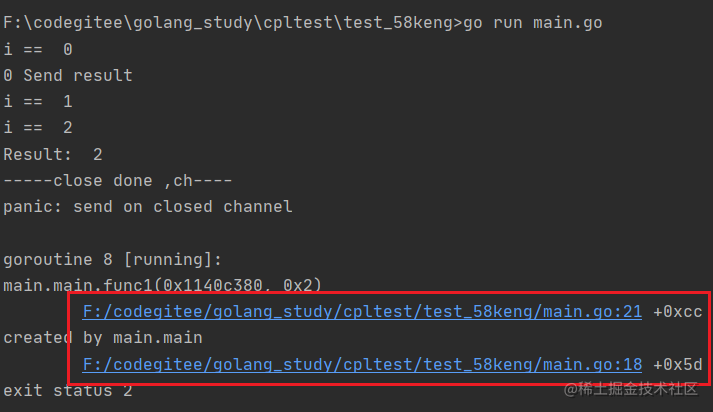``````func main() {
ch := make(chan int)
done := make(chan struct{})

for i := 0; i < 3; i++ {
go func(idx int) {
fmt.Println("i == ", idx)
select {
case ch <- (idx + 1) * 2:
fmt.Println(idx, "Send result")
case <-done:
fmt.Println(idx, "Exiting")
}
}(i)
}

fmt.Println("Result: ", <-ch)
close(done)
fmt.Println("-----close done----")
time.Sleep(3 * time.Second)
}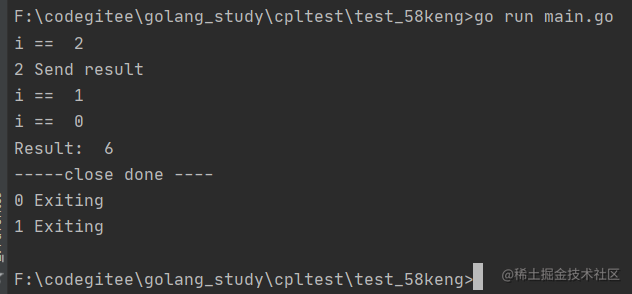``````type data struct {
num   int
key   *string
items map[string]bool
}

func (this *data) pointerFunc() {
this.num = 7
}

func (this data) valueFunc() {
this.num = 8
*this.key = "valueFunc.key"
this.items["valueFunc"] = true
}

func main() {
key := "key1"

d := data{1, &key, make(map[string]bool)}
fmt.Printf("num=%v  key=%v  items=%v\n", d.num, *d.key, d.items)

d.pointerFunc()    // 修改 num 的值为 7
fmt.Printf("num=%v  key=%v  items=%v\n", d.num, *d.key, d.items)

d.valueFunc()    // 修改 key 和 items 的值
fmt.Printf("num=%v  key=%v  items=%v\n", d.num, *d.key, d.items)
}

• valueFunc 函数中，data传的是值，是一个拷贝，并且

• num 传的是一个拷贝，因此原值没有被改变
• key 是传的指针，因此原值会被改变
• items是map ，是属于引用传递，因此也会被改变
• pointerFunc 函数中， data是传地址，因此 num原值可以被改变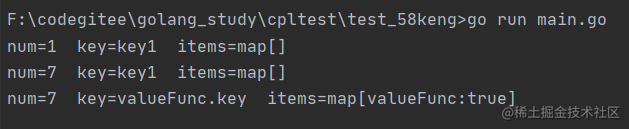## 21.关闭 HTTP 的响应体

• 请求http响应，关闭响应体的位置错误

如下代码能正确发起请求，但是一旦请求失败，变量 resp 值为 nil，造成 panic

因为 resp 为nil ， resp.Body.Close() 会是 从 nil 中 去body 然后 close，无法从空的地址中读取一段内存，因此会panic

``````// 请求失败造成 panic
func main() {
resp, err := http.Get("https://api.ipify.org?format=json")
defer resp.Body.Close()    // resp 可能为 nil，不能读取 Body
if err != nil {
fmt.Println(err)
return
}

checkError(err)

fmt.Println(string(body))
}

func checkError(err error) {
if err != nil{
log.Fatalln(err)
}
}

• 正确的做法为

先检查 HTTP 响应错误为 nil，再调用 resp.Body.Close() 来关闭响应体：

``````// 大多数情况正确的示例
func main() {
resp, err := http.Get("https://api.ipify.org?format=json")
checkError(err)

defer resp.Body.Close()    // 绝大多数情况下的正确关闭方式
checkError(err)

fmt.Println(string(body))
}

• 还会有一种重定向错误的情况，http请求返回的 resp 和 err 都不为空， 那么如何处理，有2种方式

1、 可以直接在处理 HTTP 响应错误的代码块中，直接关闭非 nil 的响应体。

2、 手动调用 defer 来关闭响应体：

``````// 正确示例
func main() {
resp, err := http.Get("http://www.baidu.com")

// 关闭 resp.Body 的正确姿势
if resp != nil {
defer resp.Body.Close()
}

checkError(err)
defer resp.Body.Close()

checkError(err)

fmt.Println(string(body))
}

resp.Body.Close() 早先版本的实现是读取响应体的数据之后丢弃，保证了 keep-alive 的 HTTP 连接能重用处理不止一个请求。

``````    _, err = io.Copy(ioutil.Discard, resp.Body) // 手动丢弃读取完毕的数据

``````    json.NewDecoder(resp.Body).Decode(&data)

## 22.关闭 HTTP 连接

• 直接设置请求变量的 Close 字段值为 true，每次请求结束后就会主动关闭连接。
• 设置`Header`请求头部选项 `Connection: close`，然后服务器返回的响应头部也会有这个选项，此时`HTTP`标准库会主动断开连接。
``````// 主动关闭连接
func main() {
req, err := http.NewRequest("GET", "http://golang.org", nil)
checkError(err)

req.Close = true

resp, err := http.DefaultClient.Do(req)
if resp != nil {
defer resp.Body.Close()
}
checkError(err)

checkError(err)

fmt.Println(string(body))
}

## 23.struct、array、slice 和 map 的值比较

``````type data struct {
num     int
fp      float32
complex complex64
str     string
char    rune
yes     bool
events  <-chan string
handler interface{}
ref     *byte
raw     byte
}

func main() {
v1 := data{}
v2 := data{}
fmt.Println("v1 == v2: ", v1 == v2)    // true
}

``````type data struct {
num    int
checks func() bool        // 无法比较
doIt   func() bool        // 无法比较
m      map[string]string    // 无法比较
bytes  []byte            // 无法比较
}

func main() {
v1 := data{}
v2 := data{}

fmt.Println("v1 == v2: ", v1 == v2)
}

invalid operation: v1 == v2 (struct containing func() bool cannot be compared)

`Go `提供了一些库函数来比较那些无法使用`==`比较的变量，比如使用 `"reflect" `包的`DeepEqual()`

``````// 比较相等运算符无法比较的元素
func main() {
v1 := data{}
v2 := data{}
fmt.Println("v1 == v2: ", reflect.DeepEqual(v1, v2))    // true

m1 := map[string]string{"one": "a", "two": "b"}
m2 := map[string]string{"two": "b", "one": "a"}
fmt.Println("v1 == v2: ", reflect.DeepEqual(m1, m2))    // true

s1 := []int{1, 2, 3}
s2 := []int{1, 2, 3}
// 注意两个 slice 相等，值和顺序必须一致
fmt.Println("v1 == v2: ", reflect.DeepEqual(s1, s2))    // true
}

``````func main() {
var b1 []byte = nil
b2 := []byte{}
fmt.Println("b1 == b2: ", reflect.DeepEqual(b1, b2))    // false
}

• `DeepEqual() `并不总适合于比较 `slice`
``````func main() {
var str = "one"
var in interface{} = "one"
fmt.Println("str == in: ", reflect.DeepEqual(str, in))    // true

v1 := []string{"one", "two"}
v2 := []string{"two", "one"}
fmt.Println("v1 == v2: ", reflect.DeepEqual(v1, v2))    // false

data := map[string]interface{}{
"code":  200,
"value": []string{"one", "two"},
}
encoded, _ := json.Marshal(data)
var decoded map[string]interface{}
json.Unmarshal(encoded, &decoded)
fmt.Println("data == decoded: ", reflect.DeepEqual(data, decoded))    // false
}

• reflect.DeepEqual() 认为空 slice 与 nil slice 并不相等，但注意 byte.Equal() 会认为二者相等：
``````func main() {
var b1 []byte = nil
b2 := []byte{}

// b1 与 b2 长度相等、有相同的字节序
// nil 与 slice 在字节上是相同的
fmt.Println("b1 == b2: ", bytes.Equal(b1, b2))    // true
}

## 24.从 panic 中恢复

``````// 错误的 recover 调用示例
func main() {
recover()    // 什么都不会捕捉
panic("not good")    // 发生 panic，主程序退出
recover()    // 不会被执行
println("ok")
}

// 正确的 recover 调用示例
func main() {
defer func() {
fmt.Println("recovered: ", recover())
}()
panic("not good")
}

## 25.在 range 迭代 slice、array、map 时通过更新引用来更新元素

``````func main() {
data := []int{1, 2, 3}
for _, v := range data {
v *= 10        // data 中原有元素是不会被修改的
}
fmt.Println("data: ", data)    // data:  [1 2 3]
}

``````func main() {
data := []int{1, 2, 3}
for i, v := range data {
data[i] = v * 10
}
fmt.Println("data: ", data)    // data:  [10 20 30]
}

``````func main() {
data := []*struct{ num int }{{1}, {2}, {3},}
for _, v := range data {
v.num *= 10    // 直接使用指针更新
}
fmt.Println(data, data, data)    // &{10} &{20} &{30}
}

## 26.旧 slice

``````// 超过容量将重新分配数组来拷贝值、重新存储
func main() {
s1 := []int{1, 2, 3}
fmt.Println(len(s1), cap(s1), s1)    // 3 3 [1 2 3 ]

s2 := s1[1:]
fmt.Println(len(s2), cap(s2), s2)    // 2 2 [2 3]

for i := range s2 {
s2[i] += 20
}
// 此时的 s1 与 s2 是指向同一个底层数组的
fmt.Println(s1)        // [1 22 23]
fmt.Println(s2)        // [22 23]

s2 = append(s2, 4)    // 向容量为 2 的 s2 中再追加元素，此时将分配新数组来存

for i := range s2 {
s2[i] += 10
}
fmt.Println(s1)        // [1 22 23]    // 此时的 s1 不再更新，为旧数据
fmt.Println(s2)        // [32 33 14]
}

## 27.跳出 for-switch 和 for-select 代码块

``````// break 配合 label 跳出指定代码块
func main() {
loop:
for {
switch {
case true:
fmt.Println("breaking out...")
//break    // 死循环，一直打印 breaking out...
break loop
}
}
fmt.Println("out...")
}

goto 虽然也能跳转到指定位置，但依旧会再次进入 for-switch，死循环。

## 28.defer 函数的参数值

``````// 在 defer 函数中参数会提前求值
func main() {
var i = 1
defer fmt.Println("result: ", func() int { return i * 2 }())
i++
}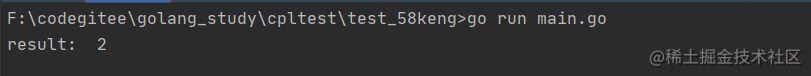## 29.defer 函数的执行时机

defer 延迟执行的函数，会在调用它的函数结束时执行，而不是在调用它的语句块结束时执行，注意区分开。

``````// 目录遍历正常
func main() {
// ...

for _, target := range targets {
func() {
f, err := os.Open(target)
if err != nil {
return    // 在匿名函数内使用 return 代替 break 即可
}
defer f.Close()    // 匿名函数执行结束，调用关闭文件资源

// 使用 f 资源
}()
}
}

## 30.更新 map 字段的值

• map 中的元素是不可寻址的

如果 map 一个字段的值是 struct 类型，则无法直接更新该 struct 的单个字段

``````// 无法直接更新 struct 的字段值
type data struct {
name string
}

func main() {
m := map[string]data{
"x": {"Tom"},
}
m["x"].name = "Jerry"
}

cannot assign to struct field m["x"].name in map

• slice 的元素可寻址：
``````type data struct {
name string
}

func main() {
s := []data{{"Tom"}}
s.name = "Jerry"
fmt.Println(s)    // [{Jerry}]
}

• 使用局部变量

最值直接用赋值的方式来进行处理

``````// 提取整个 struct 到局部变量中，修改字段值后再整个赋值
type data struct {
name string
}

func main() {
m := map[string]data{
"x": {"Tom"},
}
r := m["x"]
r.name = "Jerry"
m["x"] = r
fmt.Println(m)    // map[x:{Jerry}]
}

• 使用指向元素的 map 指针

使用的直接就是指针，无须寻址

``````func main() {
m := map[string]*data{
"x": {"Tom"},
}

m["x"].name = "Jerry"    // 直接修改 m["x"] 中的字段
fmt.Println(m["x"])    // &{Jerry}
}

``````func main() {
m := map[string]*data{
"x": {"Tom"},
}
m["z"].name = "what???"
fmt.Println(m["x"])
}

panic: runtime error: invalid memory address or nil pointer dereference

## 31.nil interface 和 nil interface 值

``````func main() {
var data *byte
var in interface{}

fmt.Println(data, data == nil)    // <nil> true
fmt.Println(in, in == nil)    // <nil> true

in = data
fmt.Println(in, in == nil)    // <nil> false    // data 值为 nil，但 in 值不为 nil
}

``````// 错误示例
func main() {
doIt := func(arg int) interface{} {
var result *struct{} = nil
if arg > 0 {
result = &struct{}{}
}
return result
}

if res := doIt(-1); res != nil {
fmt.Println("Good result: ", res)    // Good result:  <nil>
fmt.Printf("%T\n", res)            // *struct {}    // res 不是 nil，它的值为 nil
fmt.Printf("%v\n", res)            // <nil>
}
}

// 正确示例
func main() {
doIt := func(arg int) interface{} {
var result *struct{} = nil
if arg > 0 {
result = &struct{}{}
} else {
return nil    // 明确指明返回 nil
}
return result
}

if res := doIt(-1); res != nil {
fmt.Println("Good result: ", res)
} else {